Transformations: Rotations & Dilations Math Videos for Kids - Grades 6-8
1%
It was processed successfully!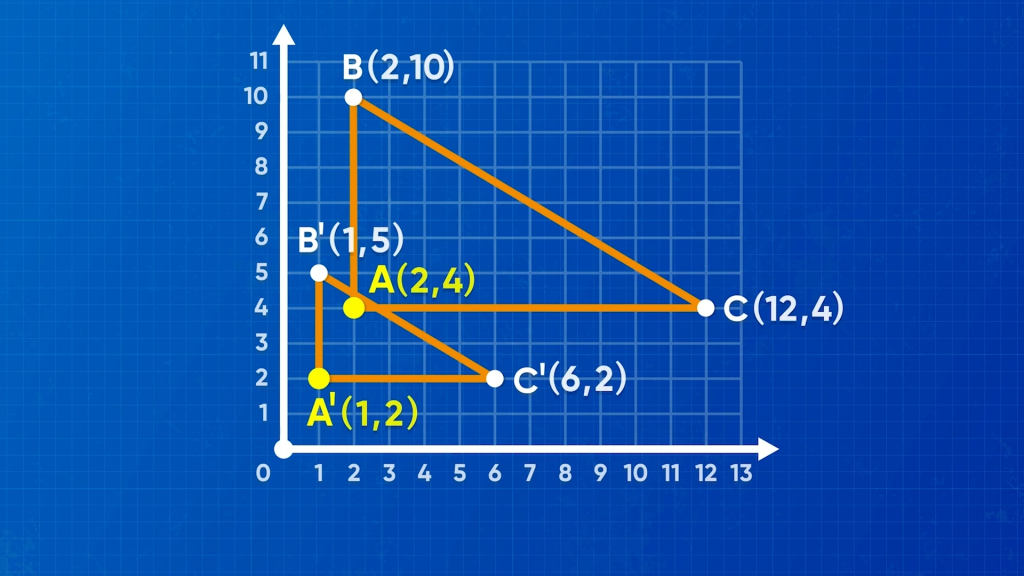WHAT ARE TRANSFORMATIONS: ROTATIONS & DILATIONS?

Just like you can translate and reflect shapes on a coordinate plane, you can also rotate and dilate them. When you rotate a shape, the resulting shape is congruent to the original. When you dilate a shape, the resulting shape is similar to the original.

To better understand translations: rotations & dilations…

WHAT ARE TRANSFORMATIONS: ROTATIONS & DILATIONS?. Just like you can translate and reflect shapes on a coordinate plane, you can also rotate and dilate them. When you rotate a shape, the resulting shape is congruent to the original. When you dilate a shape, the resulting shape is similar to the original. To better understand translations: rotations & dilations…

## LET’S BREAK IT DOWN!

### Plot values on a coordinate plane.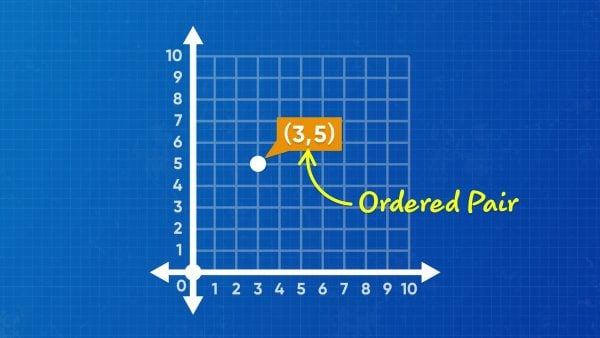All points on a coordinate plane can be described using an ordered pair where the first number is x and the second is y. An ordered pair is written (x, y). The values for x and y tell you how far the point is away from the origin on each of the two axes. For example, the point (3, 5) is 3 units to the right of the origin and 5 units above the origin. The point (3, –5) is three units to the right and 5 units down from the origin. Try this yourself: Place the following points on a coordinate plane, (3, 7), (3, –7), (5, 6), (–5, 6).

Plot values on a coordinate plane. All points on a coordinate plane can be described using an ordered pair where the first number is x and the second is y. An ordered pair is written (x, y). The values for x and y tell you how far the point is away from the origin on each of the two axes. For example, the point (3, 5) is 3 units to the right of the origin and 5 units above the origin. The point (3, –5) is three units to the right and 5 units down from the origin. Try this yourself: Place the following points on a coordinate plane, (3, 7), (3, –7), (5, 6), (–5, 6).

### What is dilation?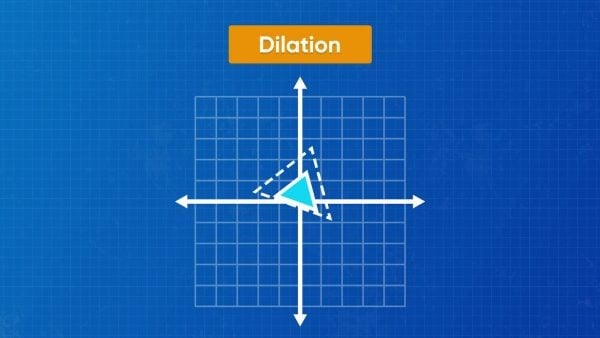A dilation is a type of transformation where you make something larger or smaller. In a dilation on a coordinate plane, a point, set of points, or figure is expanded or contracted relative to the origin. We can make a dilation by multiplying both coordinates of each point by the same number. The number we multiply by to make a dilation is called a scale factor. We can break “scale factor” down to remember what it means: “scale,” tells us how big or small something is, and “factor” is something you multiply by in a multiplication sentence. So, a scale factor is a number to multiply by to change the size of something. In a dilation, there are two figures. The first figure is called the preimage, and the second figure (which we get from performing the dilation) is called the image. If we multiply the original by a scale factor larger than 1, we perform an enlargement. If the scale factor is less than 1, we perform a reduction. Try this yourself: A preimage is dilated by a factor of 0.3. Is the dilation an enlargement or a reduction?

What is dilation? A dilation is a type of transformation where you make something larger or smaller. In a dilation on a coordinate plane, a point, set of points, or figure is expanded or contracted relative to the origin. We can make a dilation by multiplying both coordinates of each point by the same number. The number we multiply by to make a dilation is called a scale factor. We can break “scale factor” down to remember what it means: “scale,” tells us how big or small something is, and “factor” is something you multiply by in a multiplication sentence. So, a scale factor is a number to multiply by to change the size of something. In a dilation, there are two figures. The first figure is called the preimage, and the second figure (which we get from performing the dilation) is called the image. If we multiply the original by a scale factor larger than 1, we perform an enlargement. If the scale factor is less than 1, we perform a reduction. Try this yourself: A preimage is dilated by a factor of 0.3. Is the dilation an enlargement or a reduction?

### Dilate a shape.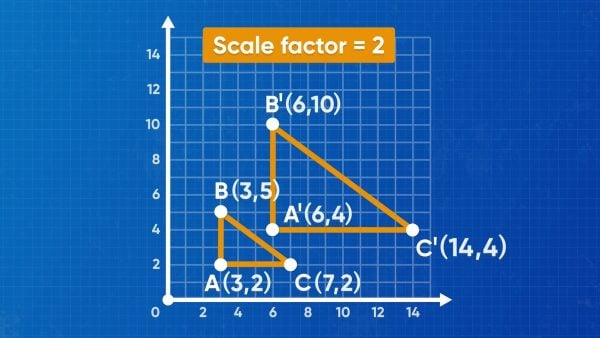In enlargement, the points in a figure move farther away from the origin, and the side lengths of the figure increase. In a reduction, the points of the figure move closer to the origin, and the side lengths in the figure decrease. For example, if we dilate the point (4, 6) by a scale factor of 2, the resulting image point is (4 × 2, 6 × 2) = (8, 12). If we dilate that same point by a scale factor of [ggfrac]1/2[/ggfrac], the resulting image point is (4 × [ggfrac]1/2[/ggfrac], 6 × [ggfrac]1/2[/ggfrac]) = (2, 3). Try this yourself: Choose three different scale factors and dilate the point (4, –8) by each of them. What do you notice?

Dilate a shape. In enlargement, the points in a figure move farther away from the origin, and the side lengths of the figure increase. In a reduction, the points of the figure move closer to the origin, and the side lengths in the figure decrease. For example, if we dilate the point (4, 6) by a scale factor of 2, the resulting image point is (4 × 2, 6 × 2) = (8, 12). If we dilate that same point by a scale factor of [ggfrac]1/2[/ggfrac], the resulting image point is (4 × [ggfrac]1/2[/ggfrac], 6 × [ggfrac]1/2[/ggfrac]) = (2, 3). Try this yourself: Choose three different scale factors and dilate the point (4, –8) by each of them. What do you notice?

### What is rotation?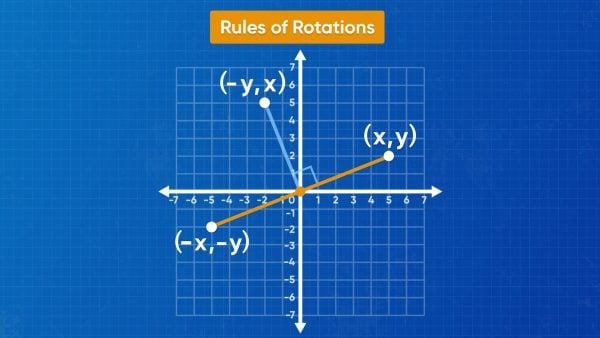Rotations are transformations where points and figures are turned on a coordinate plane. Many rotations are about the origin. In this context, “about” means around. When rotating a point about the origin, imagine a line connecting the origin to the point, and then rotate the line clockwise by the correct angle of rotation. While rotating, the length of the line between the origin and the point stays the same. If a pencil traced the path of the point around one full rotation, it would draw a circle. The common rotations are quarter turns of the circle. Since there are 360° in a circle, one-quarter turn is 90°. Try this yourself: How many degrees are there in a half turn?

What is rotation? Rotations are transformations where points and figures are turned on a coordinate plane. Many rotations are about the origin. In this context, “about” means around. When rotating a point about the origin, imagine a line connecting the origin to the point, and then rotate the line clockwise by the correct angle of rotation. While rotating, the length of the line between the origin and the point stays the same. If a pencil traced the path of the point around one full rotation, it would draw a circle. The common rotations are quarter turns of the circle. Since there are 360° in a circle, one-quarter turn is 90°. Try this yourself: How many degrees are there in a half turn?

### Rotate a shape.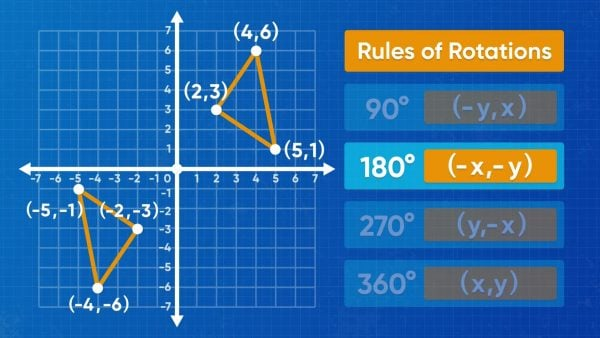You can use mapping rules to summarize the coordinates of the image points following a rotation. The mapping rule shows what the original point is, and what that point becomes to produce the image. For example, a point (x, y) undergoing a 90° clockwise rotation becomes (y, –x). Written as a mapping rule, we use an arrow to mean “becomes” or “goes to” or “maps to,” so the mapping rule for this rotation is (x,y)→(y,-x). Let’s use this rule to rotate (3, 2) 90° clockwise. First, we know that the mapping rule is (x,y)→(y,-x), so we substitute the values for x and y into the rule to get the answer (3, 2)→(2,-3). This means that the image point is (2, –3). The mapping rule for a 180° clockwise rotation is (x,y)→(-x,-y), and a 270° rotation is (x,y)→(-y,x). Since a 360° rotation is a full turn, the image and original are the same. Try this yourself: Find the image of the point (6, 4) following a 90°, 180°, 270°, and 360° clockwise rotation.

Rotate a shape. You can use mapping rules to summarize the coordinates of the image points following a rotation. The mapping rule shows what the original point is, and what that point becomes to produce the image. For example, a point (x, y) undergoing a 90° clockwise rotation becomes (y, –x). Written as a mapping rule, we use an arrow to mean “becomes” or “goes to” or “maps to,” so the mapping rule for this rotation is (x,y)→(y,-x). Let’s use this rule to rotate (3, 2) 90° clockwise. First, we know that the mapping rule is (x,y)→(y,-x), so we substitute the values for x and y into the rule to get the answer (3, 2)→(2,-3). This means that the image point is (2, –3). The mapping rule for a 180° clockwise rotation is (x,y)→(-x,-y), and a 270° rotation is (x,y)→(-y,x). Since a 360° rotation is a full turn, the image and original are the same. Try this yourself: Find the image of the point (6, 4) following a 90°, 180°, 270°, and 360° clockwise rotation.

## TRANSFORMATIONS: ROTATIONS & DILATIONS VOCABULARY

Coordinate plane
A grid made of two number lines that cross one another at zero.
x-axis
The horizontal number line, going left to right.
y-axis
The vertical number line, going down to up.
Origin
The point where the x- and y-axes cross, (0, 0).
Point
A point on a coordinate plane is represented by two numbers separated by a comma, in parentheses. In the point (x, y), "x" represents the horizontal distance from the origin, and "y" represents the vertical distance from the origin.
Transformation
The process of changing a figure or expression into its "image" by following a mathematical rule. Performing transformations is called mapping, and the rule used is called a mapping rule.
The figure or expression produced by performing a transformation on the original figure or expression.
A transformation that makes something larger or smaller. In a dilation, multiply all coordinates of the preimage by a scale factor to produce the image.
A horizontal or vertical shift of the preimage to produce the image. Horizontal shifts are achieved by adding a value to the x-coordinate of all points in the original. Vertical shifts are achieved by adding a value to the y-coordinate of all points in the original.
A mirror image of the original across the line of reflection. To reflect across the x-axis, multiply all y-coordinates by –1. To reflect across the y-axis, multiply all x-coordinates by –1. To reflect over the line y = x, switch the x- and y-coordinates. To reflect across the line y = –x, switch the x- and y-coordinates and multiply both by –1.
The factor used to dilate an original shape to its image. The scale factor is how many times larger the image is than the original. Scale factors are positive values, with scale factors less than 1 producing an image smaller than the preimage, and scale factors larger than 1 producing images larger than the preimage.
Turning a point or figure about a point to find the image. Rotations are commonly about the origin, where a point (x, y) rotated 90° clockwise has an image of (y, –x), 180° has an image of (–x, –y), and 270° has an image of (–y, x).

## TRANSFORMATIONS: ROTATIONS & DILATIONS DISCUSSION QUESTIONS

### What is a scale factor?

A scale factor is the value I multiply the coordinates of the original point by to get its image point in a dilation.

### What is the difference between an enlargement and a reduction?

Enlargements and reductions are both dilations, but an enlargement produces an image that is larger (farther from the origin) than the original, and a reduction produces an image that is smaller (closer to the origin). Enlargements have scale factors larger than 1, and reductions have scale factors between 0 and 1.

### How do you rotate a figure 90°?

First, I need to know whether to rotate clockwise or counterclockwise. The mapping rule for a clockwise 90° rotation is (x,y)→(y,-x). If I want to rotate counterclockwise, I need to find the equivalent angle: 270° clockwise is the same as 90° counterclockwise. The mapping rule for a clockwise 270° rotation is (x,y)→(-y,x).

### How can you determine what transformation was used to create an image?

First, find the corresponding vertex points. Then determine if the image is in the same orientation or a different one. If the orientation is different, look for a rotation or reflection. If the orientation is the same, check if both figures are the same size. If they are not the same size, then a dilation was used. Divide the image coordinates by the corresponding original coordinates. The answer gives the scale factor for the dilation. If there was no dilation, then the difference between the image coordinates and the original coordinates gives the translation.

### What does it mean for two 2D figures to be similar?

Two 2D figures are similar if all the corresponding angles are equal, and all side lengths share the same scale factor between them. Translations, reflections, dilations, and rotations all create similar shapes.
X

## Success

We’ve sent you an email with instructions how to reset your password.
Ok